# Cylinder and Spheres Revision Notes# Cylinder and Spheres

Volume of a Clinder =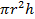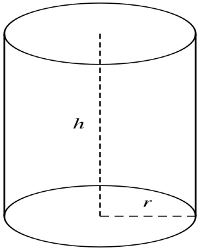r is the radius and h is the height or length of the cylinder

Curved surface Area of a cylinder= (This is the area of the rectangular part)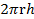Total Surface Area = + 2 (This is made up of its curved surface area plus its two circular ends)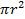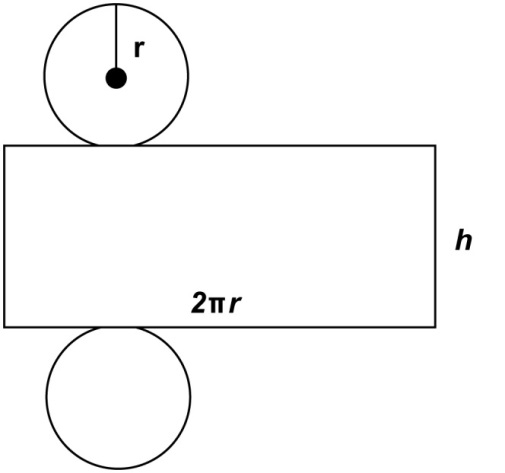This is made up of its curved surface area plus its two circular ends

Volume of a sphere= π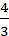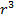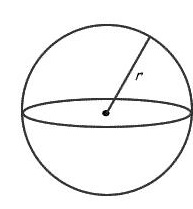Surface Area of a sphere = 4π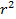Volume of a hemisphere = π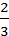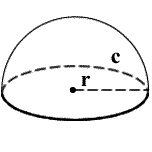Surface area of a hemisphere = 2πQuestions

1. Find the tot...